# Limited or Lots of Lemonade? - Customary Conversions

11 teachers like this lesson
Print Lesson

## Objective

SWBAT to convert liquid measures within the customary system

#### Big Idea

In this lesson students create a graphic or visual to help assist with converting within the customary system and solve a fun real world problem in a partner activity.

## Warm Up

7 minutes

Students will start today's lesson with a fluency assessment.  This assessment is from Monitoring Basic Skills Progress Second Edition: Basic Math Computation by Lynn S. Fuchs, Carol L. Hamlett, and Douglas Fuchs.

This is an assessment I have my students do each week and then graph their results.  It allows them to reflect on their learning of basic math facts, as well as using all four operations with whole numbers, and adding and subtracting unit fractions.  (It also happens to be the quietest time in my math classroom all week!!)

This is what my classroom looks like as students work on this assessment.

This is sample of a student's graph. You can also click here to hear my thoughts about this graph below.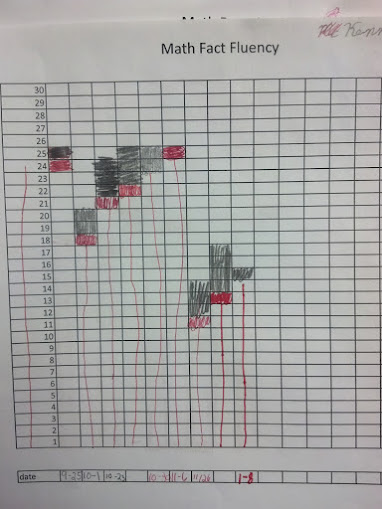Click hereto see an example of a typical fourth grade fluency decrease since students are completing the fourth grade fluency set that incorporates division, multiplication, addition, subtraction, and adding and subtracting fractions with like denominators. (At this point, students have not had many fraction lessons, thus very very students are able to complete the fraction problems)

I do not start my students with the fourth grade skills, but at this point in the year, all students are using the fourth grade set.. I chose to start them with the end of the third grade skills which covers addition, subtraction and multiplication and division of basic facts. I strongly believe in a balanced math approach, which is one reason why I also believe in common core standards.  By having a balance of building conceptual understanding, application of problems, and computational fluency, students can experience rigorous mathematics.  I want to make clear that this assessment ONLY measures basic math computation.  It is only one piece of students' knowledge. The assessments in this book, for each grade level, do not change in difficulty over the course of the year.  Therefore, a student's increase in score over the school year truly reflects improvement in the student's ability to work the math problems at that grade level.

## Concept Development

45 minutes

I begin this lesson by reviewing important measuring words from previous lessons; millimeter, centimeter, kilometer, milligram, gram, ounce, pound, milliliter, and liter. Next, I ask students what milliliters and liter measure.  (capacity)  I ask students if they know what the U.S customary system uses to measure liquid capacity. (Gallons, quarts, pints, and cups)  I lead a brief discussion about the size of each of these containers, relating them to items students know, a gallon of milk, a pint of coffee creamer, etc.

Next, I show students two different visuals or graphics that I find helpful, even for adults, when converting capacity within the customary system.   These are examples of two different graphics I show. I ask students to look for similarities and differences, but more importantly how these graphics could help them remember how many pints are in a gallon or how to convert various capacity measurements in the customary system.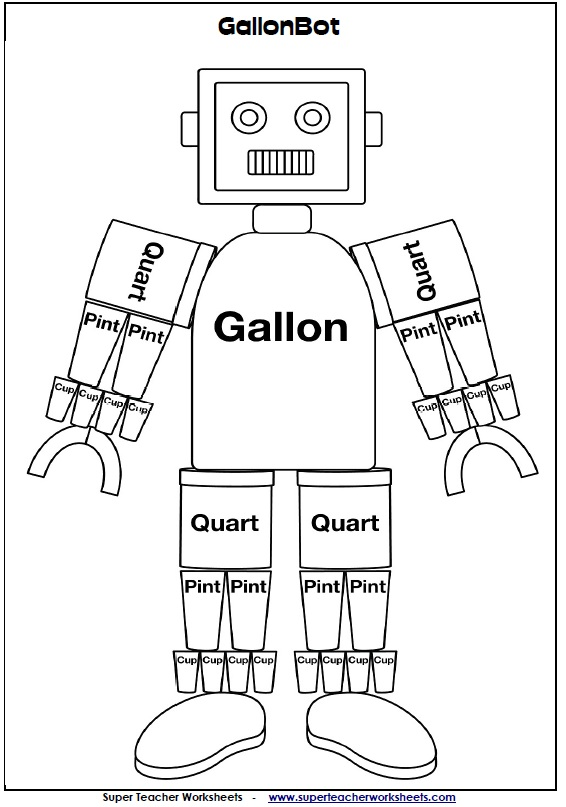(

Next, I tell students I have a problem I need their help on.  I then play this lemonade problem. - converting liquid customary and gram review problem

I play the problem twice.  The first time through, some students are still so enamored with the idea that I recorded myself in my mother's kitchen that they forget to listen for questions and important information.  The second time through, students are making sense of the problem and beginning to formulate ideas about what it is they need to do to solve the problem.  After the second time through, I make sure all students know the questions to be answered by doing a whole class share out. I write down all the questions on the board as a scaffolding tool for my students who are visual learners.  The questions the class lists are:

1. How many milligrams is equal to 246 grams?

2. How many pints is equal to 32 quarts?

3.  How many cups is equal to 32 quarts?

4.  If we make all 32 quarts of lemonade, will we have enough lemonade for every student in fourth grade?

5.  If we don't have enough, how many more cups do we need?  If we have enough, how many extra cups will we have left over?

Then students work with their learning partner to solve all five questions.  The following photos show two different student work samples.  You can see in this first example, the student has written all of the questions to answer at the top of his/her page.  One interesting thing about this paper is that this student does not appear to be using multiplication to solve the question about pints. You can see that this student has drawn 32 "Q's" to represent the quarts.  When I watched this student, the student then counted by twos to find the amount of pints in 32 quarts. This is important for me to know so that I can make sure during the sharing time that this student is able to hear other students more sophisticated and efficient methods of using multiplication.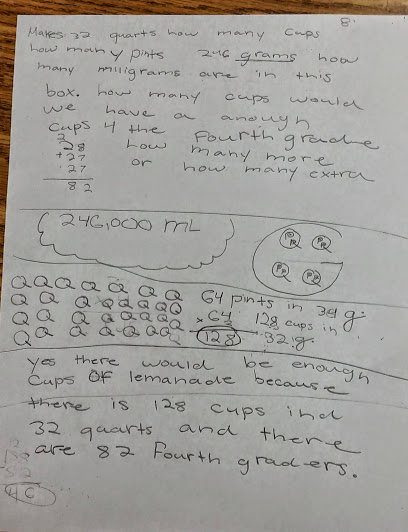Here is another work sample. Note in this sample, the students has drawn the gallon visual on his/her paper as a reference to aid in converting the various units in this problem.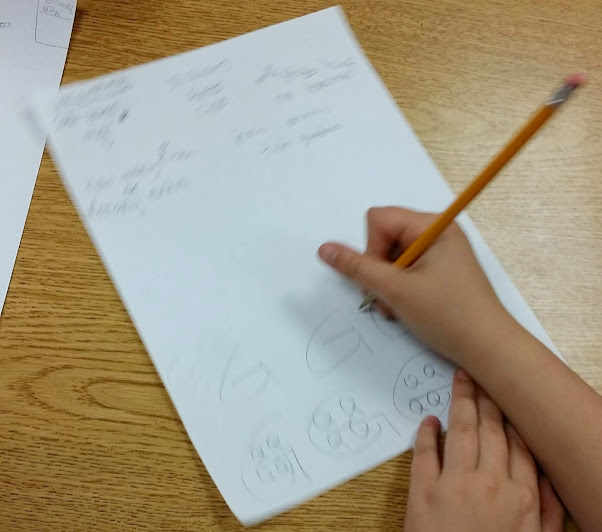Listen in as this student tells how she is counting to determine her conversions. Notice the similarity to the first photo.  Both of these students will benefit from hearing other students strategies.

As you can see from above, there is a wide variety of understanding in my classroom in terms of what strategies are most efficient. Because of this, I designed this lesson to have a bonus question for students to work on while they waited for all groups to finish. The bonus question is: How many boxes of this lemonade should I buy if every student at Four Georgians had one cup?  Students work on this last question while other groups work to finish the first five questions.

For homework, I assign students the task of creating a graphic representation of gallons, quarts, pints, and cups that makes sense to them. While I really like my graphic and think it makes sense, I also know that students must own this knowledge for themselves and let it make sense to them.

Students were very creative in their own designs.  Some of student designs truly took on personalities reflective of that student.  Here are some example.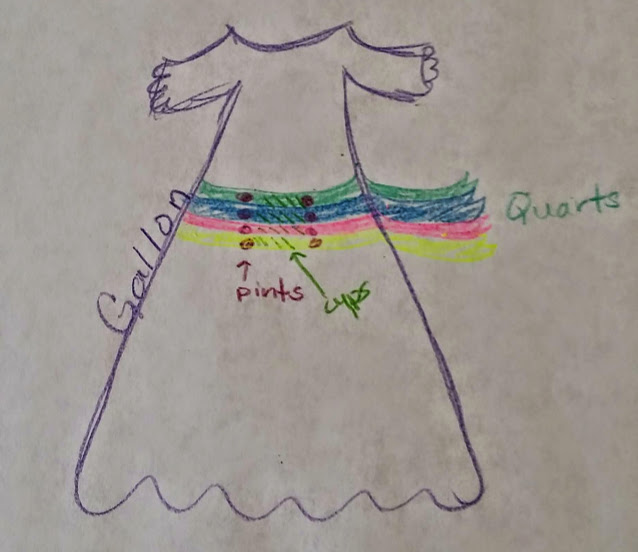## Wrap Up

12 minutes

One of the important parts of this lesson, that should NOT be left out, is this wrap up. For this wrap up, my students participate in an activity I call "Pass and Read".

In Pass and Read, students stand in a silent circle with their papers or work. Student are not allowed to talk during the initial phase of the pass and read. One my signal,( I say PASS) students pass their paper one person to the left.  I may stop at this point and say READ, or I may say PASS again.  If I say READ, students stop and read the new paper they are now holding. They are not asking questions out loud at this point, simply looking at the work on the paper.  Their job is to analyze and evaluate the work. Students should be thinking to themselves, Is this correct?  Is this similar or different to mine?  What would I ask this person? What would I want clarification on

I give students only a few seconds, 15 to 30 to look at the paper. Then I say PASS.  Students will continue to pass the paper to the left. I may say something like this: PASS, PASS, PASS, PASS, PASS, READ!  In this example, students, in unison, would pass each new paper to their left five times before they stopped to read a paper.

I continue in this way until all papers have gone around the circle and end up back to it's owner.

The second phase of the Pass and Read, is important. This is when students ask the questions they had to the group. Students share things they noticed and wondered as they looked at papers. It is in this phase that I as a teacher really need to help make math connections and relationships for students. For example, in the concept development portion of this lesson, I saw students counting on and counting by two in order to calculate how many pints were in 32 quarts.  I ask a student who displayed this strategy to share his/her ideas. Then I purposely call on another student that I observed using multiplication.  I ask all students to think about how the two strategies are similar, different, and efficient.  Many students at this point, begin to realize they could use multiplication instead of counting on or counting by two's or fours.  This is crucial for students as they make connections between strategies.  If I had stopped students during their work, and said, "No, you need to multiply," students have little understanding as to why they should. It is only after they see and hear from other students work that they begin to own this knowledge for themselves.